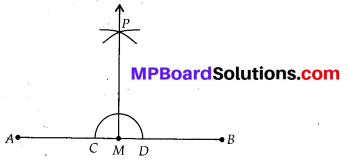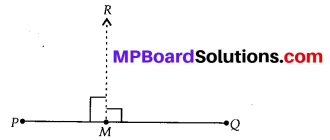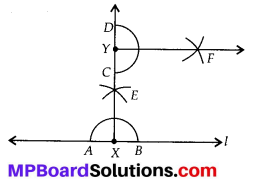## MP Board Class 6th Maths Solutions Chapter 14 Practical Geometry Ex 14.4

Question 1.
Draw any line segment $$\overline{A B}$$. Mark any point M on it. Through M, draw a perpendicular to $$\overline{A B}$$. (use ruler and compasses)
Solution:
Steps of construction:(i) Draw $$\overline{A B}$$.
(ii) With M as centre and a convenient radius, draw an arc intersecting $$\overline{A B}$$ at two points C and D.
(iii) With C and D as centres and a radius greater than MC, draw two arcs, which cut each other at P.
(iv) Join PM. Then PM is perpendicular to AB through the point M.Question 2.
Draw any line segment $$\overline{P Q}$$. Take any point R not on it. Through R, draw a perpendicular to $$\overline{P Q}$$. (use ruler and set-square)
Solution:
Steps of construction :(i) Draw any line segment $$\overline{P Q}$$.
(ii) Place a set-square on $$\overline{P Q}$$ such that one arm’ of its right angle aligns along $$\overline{P Q}$$.
(iii) Place a ruler along the edge opposite to the right angle of the set-square.
(iv) Hold the ruler fixed. Slide the set square along the ruler till the point R touches the other arm of the set square.
(v) Join RM along the edge through R meeting $$\overline{P Q}$$ at M. Then RM⊥PQ.Question 3.
Draw a line l and a point X on it. Through X, draw a line segment $$\overline{X Y}$$ perpendicular to l. Now draw a perpendicular to $$\overline{X Y}$$ at Y. (use ruler and compasses)
Solution:
Steps of construction :(i) Draw a line ‘V and take point X on it.
(ii) With X as centre and a convenient radius, draw an arc intersecting the line ‘l’ at two points A and B.
(iii) With A and B as centres and a radius greater than XA, draw two arcs, which cut each other at E.
(iv) Join XE and produce it to Y. Then XY is perpendicular to ‘l’.
(v) With Y as centre and a convenient radius, draw an arc intersecting XY at two points C and D.
(vi) With C and D as centre and radius greater than YD, draw two arcs which cut each other at F.
(vii) Join YF, then YF is perpendicular to XY at Y.